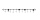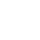# Mixed fractions calculator

The calculator performs basic and advanced operations with mixed numbers, fractions, integers, decimals. Mixed fractions are also called mixed numbers. A mixed fraction is a whole number and a proper fraction combined, i.e. one and three-quarters. The calculator evaluates the expression or solves the equation with step-by-step calculation progress information. Solve problems with two or more mixed numbers fractions in one expression.

The calculator performs basic and advanced operations with mixed numbers, fractions, integers, decimals. Mixed fractions are also called mixed numbers. A mixed fraction is a whole number and a proper fraction combined, i.e. one and three-quarters. The calculator evaluates the expression or solves the equation with step-by-step calculation progress information. Solve problems with two or more mixed numbers fractions in one expression.

## Result:

### 7/2 = 7/2 = 3 1/2 = 3.5

Spelled result in words is three and one half (or seven halfs).

#### Examples:

sum of two mixed numbers: 1 3/4 + 2 3/8
addition of three mixed numbers: 1 3/8 + 6 11/13 + 5 7/8
addition of two mixed numbers: 2 1/2 + 4 2/3
subtracting two mixed numbers: 7 1/2 - 5 3/4
multiplication of mixed numbers: 3 3/4 * 2 2/5
comparing mixed numbers: 3 1/4 2 1/3
changing improper fraction to mixed number: 9/4
What is 3/4 as a mixed number: 3/4
subtracting mixed number and fraction: 1 3/5 - 5/6
sum mixed number and an improper fraction: 1 3/5 + 11/5

## Mixed fractions in word problems:

• Mixed numbersRewrite mixed numbers, so the fractions have the same denominator: 5 1/5 - 2 2/3
• Between two mixedWhat is the rational number between 2 1/4 and 2 4/5?
• Mixed divided with fracThere are 2 2/3 pizzas. How many people are sharing when each has 2/3 of pizza?
• Adding mixed numbersAdd this two mixed numbers: 1 5/6 + 2 2/11=
• Mixed2improperWrite the mixed number as an improper fraction. 166 2/3
• Mixed with percentagesCalculate 33 1/3% of 570.
• Divide 11Divide the product of 4 and 5/8 by 1 1/2. Write your answer as a mixed number.
• Difference mixed fractionsWhat is the difference between 4 2/3 and 3 1/6?
• Addition of mixed numeralsAdd two mixed fractions: 2 4/6 + 1 3/6
• To improper fractionChange mixed number to improper fraction a) 1 2/15 b) -2 15/17
• Add sub fractionsWhat is 4 1/2+2/7-213/14?
• Cubic inches to cups5-3/8" x 4" x 2-5/8" container. How many equals cups?
• WillieWillie collected 6 2/3 pails of water. How many big containers can she fill each container contains 2 2/9 of a pail?
• Adding mixed 3Why does 1 3/4 + 2 9/10 equal 4.65? How do you solve this?
• Clock mathematicsIf it is now 7:38 pm, what time will it be in 30,033,996,480 minutes from now?
• Two pizzasJacobs mom bought two whole pizzas. He ate 2/10 of the pizza and his dad ate 1 1/5. How much is left.
• An airplaneAn airplane flies 1440 km in 2 1/4 hours. What is its average speed in km per hour?